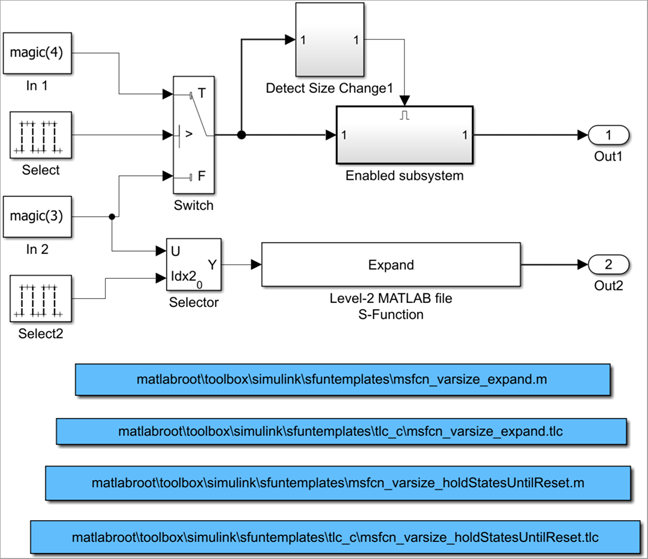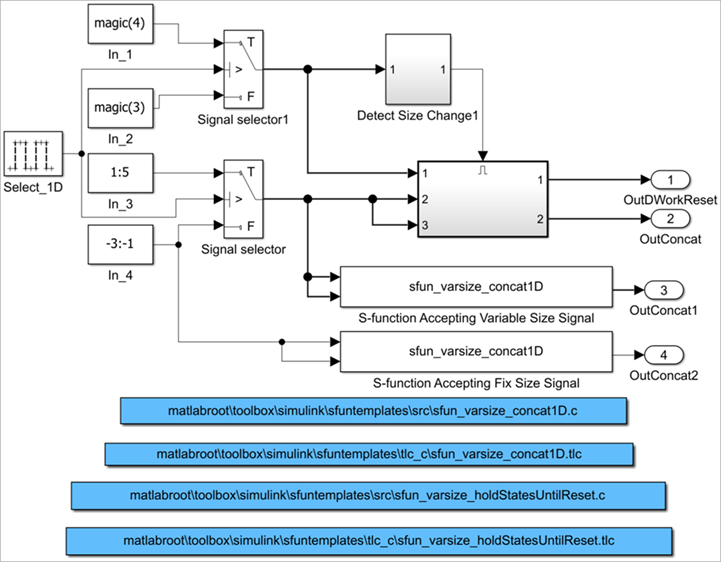## S-Functions Using Variable-Size Signals

### Level-2 MATLAB S-Function with Variable-Size Signals

Both Level-2 MATLAB® S-Functions and C S-Functions support variable-size signals when you set the DimensionMode for the output port to `Variable`. You also need to consider the current dimension of the input and output signals in the input and output update methods.

To open this example model, in the MATLAB Command Window, type:

`msfcndemo_varsize`The Enabled subsystem includes a Level-2 MATLAB S-Function which shows how to implement a block that holds its states until reset. Because this block contains states and delays the input signal, the input size can change only when a reset occurs.

The Expand block is a Level-2 MATLAB S-Function that takes a scalar input and outputs a vector of length indicated by its input value. The output is by `1:n` where `n` is the input value.

### C S-Function with Variable-Size Signals

To open this example model, in the MATLAB Command Window, type:

`sfcndemo_varsize`The enabled subsystems have two S-Functions:

• sfun_varsize_holdStatesUntilReset is a C S-Function that has states and requires its DWorks vector to reset whenever the sizes of the input signal changes.

• sfun_varsize_concat1D is a C S-function that implements the concatenation of two unoriented vectors. You can use this function within an enabled subsystem by itself.